• 灰色邓氏关联度分析 % P12 The Study on the Grey Relational Degree and Its Application function rl = gld_deng(x) s size (x; len s(2; num s(1; ro 0?5; fori = 1: num x (ir : ) = x (if ? /x (iz 1; end dx...
• 灰色系统理论提出了对各子系统进行灰色关联度分析的概念，意图透过一定的方法，去寻求系统中各子系统（或因素）之间的数值关系。因此，灰色关联度分析对于一个系统发展变化态势提供了量化的度量，非常适合动态历程...
• 邓聚龙提出的灰色关联分析算法的实现过程。可以在此基础上进行二次开发。
• 参加数学建模时自己编写的一个灰色关联分析法的函数封装，里面都有注释说明。
• 灰色关联度的基础代码，灰色关联度的基础代码，灰色关联度的基础代码。
• 详细的代码，提供学习。详细的代码，提供学习。详细的代码，提供学习。详细的代码，提供学习。详细的代码，提供学习。
• 灰色关联度分析（Grey Relation Analysis，GRA），是一种多因素统计分析的方法。简单来讲，就是在一个灰色系统中，我们想要了解其中某个我们所关注的某个项目受其他的因素影响的相对强弱，再直白一点，就是说：我们...


文章目录
1.释名2.举例3.操作步骤与原理详解4.总结5.附录：MATLAB代码

1.释名
灰色关联度分析（Grey Relation Analysis，GRA），是一种多因素统计分析的方法。简单来讲，就是在一个灰色系统中，我们想要了解其中某个我们所关注的某个项目受其他的因素影响的相对强弱，再直白一点，就是说：我们假设以及知道某一个指标可能是与其他的某几个因素相关的，那么我们想知道这个指标与其他哪个因素相对来说更有关系，而哪个因素相对关系弱一点，依次类推，把这些因素排个序，得到一个分析结果，我们就可以知道我们关注的这个指标，与因素中的哪些更相关。
（ note ： 灰色系统这个概念的提出是相对于白色系统和黑色系统而言的。这个概念最初是由控制科学与工程的教授邓聚龙提出的。按照控制论的惯例，颜色一般代表的是对于一个系统我们已知的信息的多少，白色就代表信息充足，比如一个力学系统，元素之间的关系都是能够确定的，这就是一个白色系统；而黑色系统代表我们对于其中的结构并不清楚的系统，通常叫做黑箱或黑盒的就是这类系统。灰色介于两者之间，表示我们只对该系统有部分了解。）
2.举例
为了说明灰色关联度分析的应用场景，我们利用下图进行说明：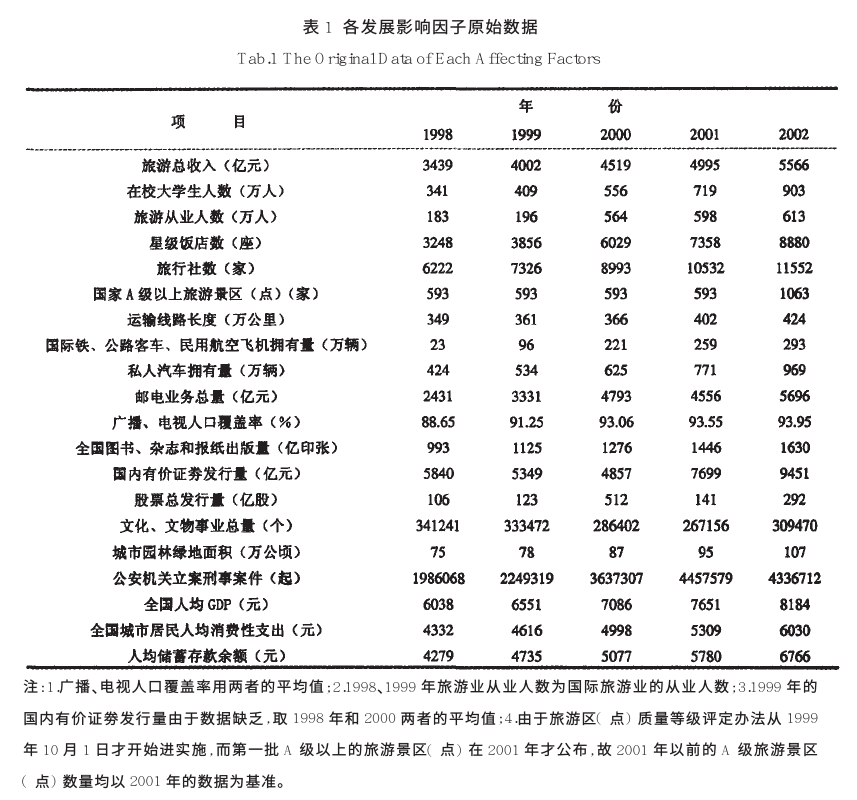该图研究的内容是旅游业发展的影响因子，看该表格，第一行为五年的旅游总收入，代表着旅游业发展的程度，而下面的这些要素就是我们需要分析的因子，比如在校大学生数，旅行社数，星级饭店数，A级景区数等等。最终目的是要得到一个排序，从而说明这些因子对旅游总收入的关联性的程度。
3.操作步骤与原理详解
（1） 确立母序列 （参考序列，在上面栗子中就是1998~2002年的旅游总收入序列）和子序列（比较序列，也就是需要确立顺序的因素序列，上栗中的除了第一行以外的所有因素都可以作为参考序列） 为了后面的表述方便，这里统一一下notation：
我们用x_i(k)表示第i个因素的第k个数值
用上面的栗子来说
比如第一个因素是在校大学生人数，那么x_1(1)就表示在校大学生人数在1998年的取值，也就是341，x_1(2)就是1999年的取值，
而x_2(1)就是表示旅游从业人数在1998年的数值。以此类推。
我们用x_0(k)表示母序列，i≥1的表示子序列，也就是要分析的要素的序列。
如果不写括号，比如x_i ，就代表这个元素的整个序列，也就是向量 x_i = [x_i(1), x_i(2), ... , x_i(n)]
n为每个向量的维度，也就是每个元素的特征的数量，在上栗中，n就是5，因为有五年的数据，代表五维向量。
以下所有表述都用该notation表示。

详解： 这个就是我们任务的目的（找到子序列和参考序列的关联程度） （2）归一化，或者叫 无量纲化。 详解： 因为我们的这些要素是不同质的东西的指标，因此可能会有的数字很大有的数字很小，但是这并不是由于它们内禀的性质决定的，而只是由于量纲不同导致的，因此我们需要对它们进行无量纲化。这个操作一般在数据处理领域叫做归一化（normalization），也就是减少数据的绝对数值的差异，将它们统一到近似的范围内，然后重点关注其变化和趋势。 如下图所示，这是上面表格中前3个元素随年的变化曲线，以及作为母序列的旅游总收入：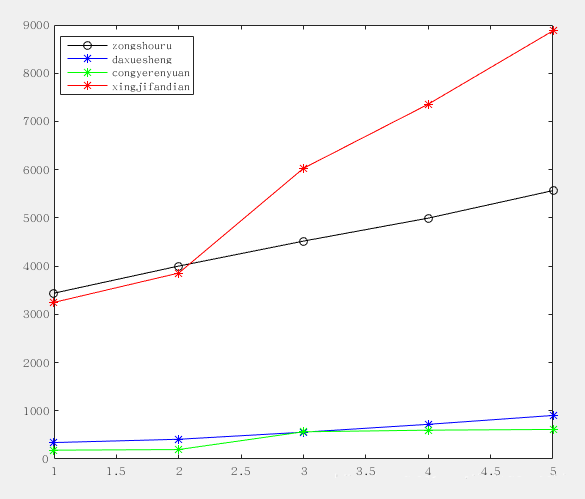可以看到，有两个曲线绝对数值很大，而另外两个很小，如果不做处理必然导致大的数值的影响会”淹没“掉小数值的变量的影响。
所以我们要对数据进行归一化处理，主要方法有如下几个： （1）初值化： 顾名思义，就是把这一个序列的数据统一除以最开始的值，由于同一个因素的序列的量级差别不大，所以通过除以初值就能将这些值都整理到1这个量级附近。
公式： x_i(k)' = x_i(k) / x_i(1)   i = 1,...,m, k = 1,...,n
(m为因素个数，n为每个因素的数据维度，仍如上栗，n=5，m=3（我们只看前三个因素，
就是曲线图里画的这三种，和旅游总收入的关联，数据维度为5，即五年）)

（2） 均值化： 顾名思义，就是把这个序列的数据除以均值，由于数量级大的序列均值比较大，所以除掉以后就能归一化到1的量级附近。
公式： x_i(k)' = x_i(k) / ( mean(x_i) )        （除以均值）
其中 ： mean(x_i) = (1/n) sum_k=1^n (x_i(k))     （求第i个因素序列的均值）

用初值化进行归一化，得到的结果如下图：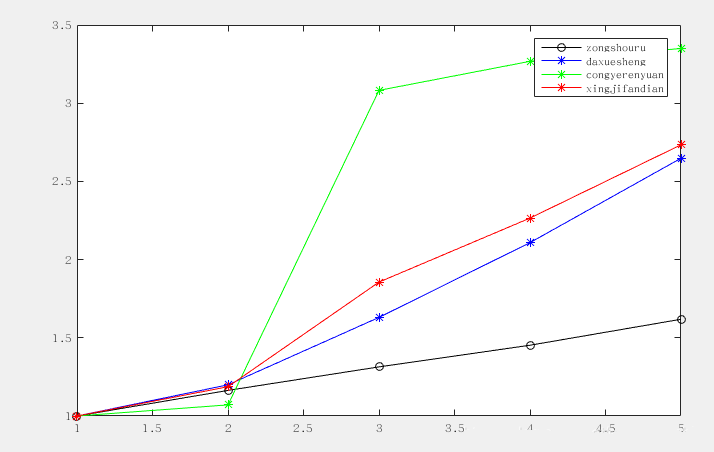可以看到，归一化以后的数据，量级差别变小了，这是为了后面提供铺垫，因为我们关注的实际上是曲线的形状的差异，而不希望绝对数值对后面的计算有影响。 （3）计算灰色关联系数 先放上公式：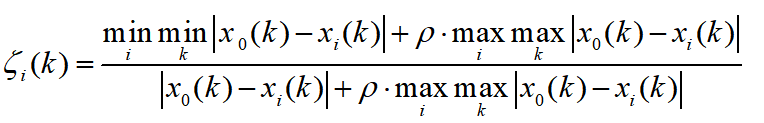详解： 首先，我们把i看做固定值，也就是说对于某一个因素，其中的每个维度进行计算，得到一个新的序列，这个序列中的每个点就代表着该子序列与母序列对应维度上的关联性（数字越大，代表关联性越强）。 仔细观察这个公式，rho是一个可调节的系数，取值为(0,1)，大于零小于一，这一项的目的是为了调节输出结果的差距大小，我们放在后面讲。我们先假设把rho取成0，那么，这个式子就变成了
pseudo_zeta_i(k) = min min |x_0(k) - x_i(k)| / |x_0(k) - x_i(k)|
= constant / |x_0(k) - x_i(k)|

我们看上面这个式子，可以发现，分子上这个数值，对于所有子序列来说都是一样的（，分子上这个数实际上就是所有因素的所有维度中，与母序列（参考序列，即我们要比较的序列）距离最近的维度上的距离。为什么要这样做呢？这样来想，假如我们没有进行归一化，或者不是用的初值化，而是用的均值化或者其他方法，可能会导致曲线之间，也就是母序列和各个子序列之间仍然有一段距离，那么这个距离最小值与下面的每个维度的距离相除，实际上也可以看成是一种取消量纲的手段。对于所有子序列，这个分子是相同的，所以实际上，这个系数pseudo_zeta是与第k个维度上，子序列与母序列的距离（差的绝对值，通常叫做l1范数（l1-norm））成反比，也就是说，这两个数距离越远，我们认为越不相关，这是符合直觉的。
当然，如果用了初值化归一化数据，如上面的图2所示，min min |x_0(k) - x_i(k)| 对每个i都会变成0，这样就不好了，因为这样一来，所有的zeta_i(k)都成了0，是无意义的。所以这时候我们就看到后面的 rho max max这一项的作用了。这一项对于每个i来说也是一个不变的常数constant，所以可以理解为给上面那个式子的分子分母同时加上某个数值，如下所示：
zeta_i(k) = (aconstant + bconstant) / (|x_0(k) - x_i(k)| + bconstant)

这样做的目的是什么呢？ 我们举个栗子： 对于两个分数： 1/5 和 1/4 ，它们的分子一样，分母相差为1，这时候他们的值相差1/20，也就是0.05，这就是没有＋rho max max那一项的情况，分子相同，分母的差代表着与参考序列的距离。 如果我们给他们分子分母同时加上20，那么就是21/25和21/24，它们相差为0.035，可以看到，加入这一项会导致同样的距离的点的系数差，会因为计算而变小。很显然地，rho取得越大，不同zeta系数的差距就越小。
另外，由于分子上是min min，也就是距离的全局最小值，这就导致下面的分母必然大于分子（不考虑 rho max max 项），而且，如果分母非常大，曲线距离非常远，那么，zeta接近0； 相反，如果x_i和x_0在所有维度上的差完全一样，那么分数的值就是1。这样zeta取值范围就是0~1之间，0表示不相关，1表示强关联性。这也符合认知。考虑上rho max max 项之后，我们知道对于一个真分数，分子分母都加一个同样的值，仍然是真分数（实际上是一个添加溶质的溶液的问题）。也就是说，仍然是0到1。
总结来说，rho是控制zeta系数区分度的一个系数，rho取值0到1， rho越小，区分度越大，一般取值0.5较为合适。zeta关联系数取值落在0到1之间。
接上栗，我们对上述三个子序列做出关联系数zeta的序列，结果如下：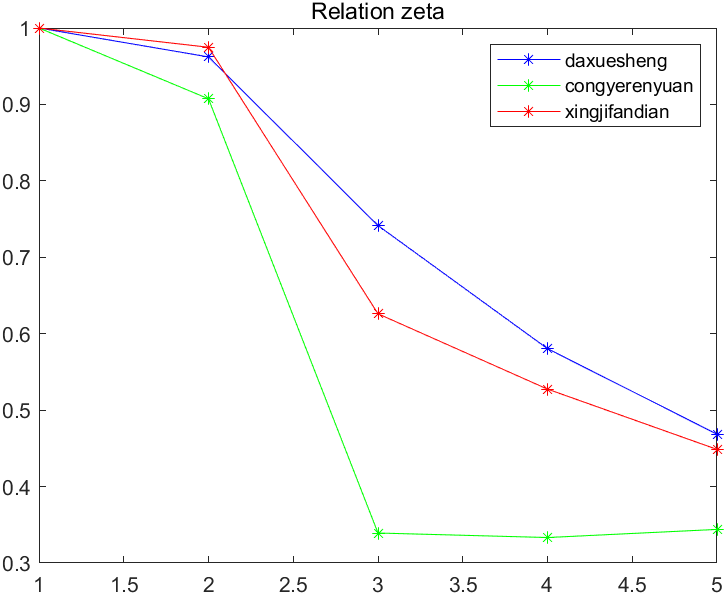其实从这个图中已经可以看出，大学生这一因素对旅游也的相关性普遍要高一些，从业人员相对影响少一些。星级饭店的数量居中。 （4）计算关联系数均值，形成关联序 根据上图其实已经可以看出大概的趋势，但是这只是因为这个恰好所有维度上的趋势比较一致，实际上，我们得到zeta关联系数的值以后，应该对每个因素在不同维度上的值求取均值，换句话说，也就是对于上面那些zeta 的曲线，同一个颜色的求取均值。结果如下：
>> mean(zeta_1)
ans =
0.7505
>> mean(zeta_2)
ans =
0.5848
>> mean(zeta_3)
ans =
0.7154

可以看到，根据关联系数大小，排序结果为：
大学生人数 ＞ 星级饭店数量 ＞ 从业人员人数
4.总结
GRA算法本质上来讲就是提供了一种度量两个向量之间距离的方法，对于有时间性的因子，向量可以看成一条时间曲线，而GRA算法就是度量两条曲线的形态和走势是否相近。为了避免其他干扰，凸出形态特征的影响，GRA先做了归一化，将所有向量矫正到同一个尺度和位置，然后计算每个点的距离。最后，通过min min 和max max 的矫正，使得最终输出的结果落在0到1之间，从而符合系数的一般定义。rho调节不同关联系数之间的差异，换句话说，就是输出的分布，使其可以变得更加稀疏或者紧密。以数学角度要言之，该算法即度量已归一化的子向量与母向量的每一维度的l1-norm距离的倒数之和，并将其映射到0～1区间内，作为子母向量的关联性之度量的一种策略。
5.附录：MATLAB代码
% Grey relation analysis

clear all
close all
clc

zongshouru = [3439, 4002, 4519, 4995, 5566];
daxuesheng = [341, 409, 556, 719, 903];
congyerenyuan = [183, 196, 564, 598, 613];
xingjifandian = [3248, 3856, 6029, 7358, 8880];

% define comparative and reference
x0 = zongshouru;
x1 = daxuesheng;
x2 = congyerenyuan;
x3 = xingjifandian;

% normalization
x0 = x0 ./ x0(1);
x1 = x1 ./ x1(1);
x2 = x2 ./ x2(1);
x3 = x3 ./ x3(1);

% global min and max
global_min = min(min(abs([x1; x2; x3] - repmat(x0, [3, 1]))));
global_max = max(max(abs([x1; x2; x3] - repmat(x0, [3, 1]))));

% set rho
rho = 0.5;

% calculate zeta relation coefficients
zeta_1 = (global_min + rho * global_max) ./ (abs(x0 - x1) + rho * global_max);
zeta_2 = (global_min + rho * global_max) ./ (abs(x0 - x2) + rho * global_max);
zeta_3 = (global_min + rho * global_max) ./ (abs(x0 - x3) + rho * global_max);

% show
figure;
plot(x0, 'ko-' )
hold on
plot(x1, 'b*-')
hold on
plot(x2, 'g*-')
hold on
plot(x3, 'r*-')
legend('zongshouru', 'daxuesheng', 'congyerenyuan', 'xingjifandian')

figure;
plot(zeta_1, 'b*-')
hold on
plot(zeta_2, 'g*-')
hold on
plot(zeta_3, 'r*-')
title('Relation zeta')
legend('daxuesheng', 'congyerenyuan', 'xingjifandian')

展开全文• ## 灰色关联分析+Matlab代码实现

万次阅读 多人点赞 2020-12-11 10:25:01
3.灰色关联分析代码实现(Matlab) 学习时间： 2020.12.11 学习产出： 1.灰色关联分析基本思想 2.运用灰色关联分析的基本步骤 ①确定分析数列 母序列(又称参考数列，母指标)：能反映系统行为特征的数据序列——>...
学习内容：
1.灰色关联分析的基本思想 2.运用灰色关联分析的基本步骤 3.灰色关联分析代码实现(Matlab)

学习时间：
2020.12.11

学习产出：
1.灰色关联分析基本思想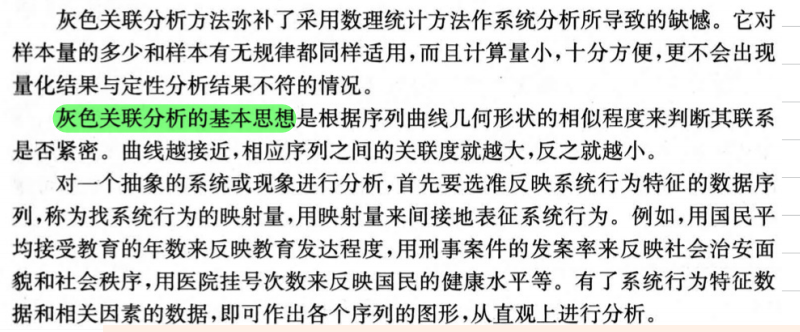2.运用灰色关联分析的基本步骤 ①确定分析数列 母序列(又称参考数列，母指标)：能反映系统行为特征的数据序列——>类似与因变量Y 子序列(又称比较数列，子指标)：影响系统行为的因素组成的数据序列——>类似与自变量X ②对变量进行预处理(去量纲，缩小变量范围，简化计算)，先求出每个指标列的均值，再用该指标列的每一个元素都除以该指标列的均值 ③用子序列中每一个元素减去对应母序列中同一行的那个元素，并取绝对值，由此得到一个新矩阵new_X。 记a为矩阵中的最小元素，b为矩阵中的最大元素，分辨系数ro通常为0.5，那么每一个元素对应母序列的关联系数为 a+ro*b./(new_X+ro*b) ,然后，我们再对得到的关联系数矩阵求每一列均值，得到的最后结果gamma就是每一个指标对于母序列的灰色关联度
3.灰色关联分析代码实现(Matlab) ①应用一：分析产业对GDP的影响程度 数据：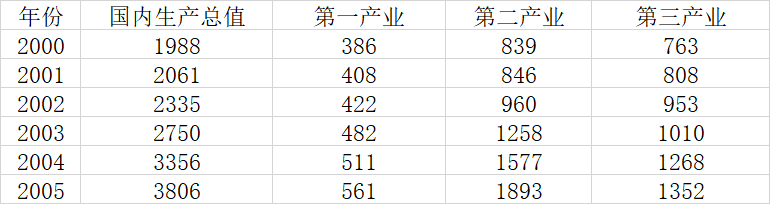%% 应用一：分析产业对GDP的影响程度
clear;clc;
r = size(data,1);
c = size(data,2);
%第一步，对变量进行预处理，消除量纲的影响
avg = repmat(mean(data),r,1);
data = data./avg;
%定义母序列和子序列
Y = data(:,1); %母序列
X = data(:,2:c); %子序列
Y2 = repmat(Y,1,c-1); %把母序列向右复制到c-1列
absXi_Y = abs(X-Y2)
a = min(min(absXi_Y)) %全局最小值
b = max(max(absXi_Y)) %全局最大值
ro = 0.5; %分辨系数取0.5
gamma = (a+ro*b)./(absXi_Y+ro*b) %计算子序列中各个指标与母序列的关联系数
disp("子序列中各个指标的灰色关联度分别为：");
ans = mean(gamma)

输出结果为：
子序列中各个指标的灰色关联度分别为：

ans =

0.5084    0.6243    0.7573

②应用二：灰色关联分析评价河流情况 数据：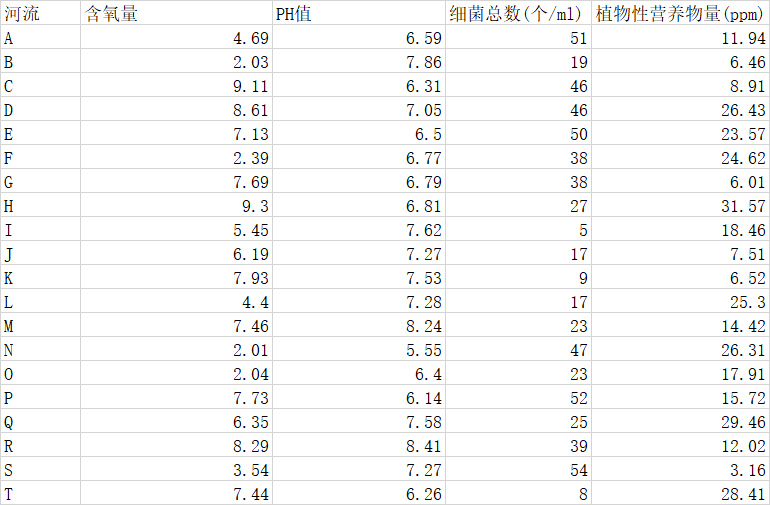%应用二：灰色关联分析评价河流情况
clear;clc;
%获取行数列数
r = size(X,1);
c = size(X,2);
%首先，把我们的原始指标矩阵正向化
%第二列中间型--->极大型
middle = input("请输入最佳的中间值：");
M = max(abs(X(:,2)-middle));
for i=1:r
X(i,2) = 1-abs(X(i,2)-middle)/M;
end
%第三列极小型--->极大型
max_value = max(X(:,3));
X(:,3) = abs(X(:,3)-max_value);
%第四列区间型--->极大型
a = input("请输入区间的下界：");
b = input("请输入区间的下界：");
M = max(a-min(X(:,4)),max(X(:,4))-b);
for i=1:r
if (X(i,4)<a)
X(i,4) = 1-(a-X(i,4))/M;
elseif (X(i,4)<=b&&X(i,4)>=a)
X(i,4) = 1;
else
X(i,4) = 1-(X(i,4)-b)/M;
end
end
disp("正向化后的矩阵为：");
disp(X);
%把正向化后的矩阵进行预处理，消除量纲的影响
avg = repmat(mean(X),r,1);
new_X = X./avg;
%将预处理后的矩阵每一行的最大值取出，当成母序列(虚构的)
Y = max(X,[],2);
%计算各个指标和母序列的灰色关联度
%先把new_X矩阵所有元素都减去母序列中同行的元素，并取绝对值
Y2 = repmat(Y,1,c);
new_X = abs(new_X-Y2);
a = min(min(new_X)); %全矩阵最小值
b = max(max(new_X)); %全矩阵最大值
ro = 0.5;
new_X = (a+ro*b)./(new_X+ro*b);
disp("各个指标对于母序列的灰色关联度为：");
gamma = mean(new_X)
%计算各个指标的权重
disp("各个指标的权重为：");
weight = gamma./(sum(gamma,2))
%-------------------------------------------------------------------------------------------------------
%继续TOPSIS的步骤：对正向化后的矩阵X进行标准化（原矩阵除以每一列元素平方之和的开方）
temp1 = X.*X;               %先让每每一个元素平方
temp2 = sum(temp1);         %再对每一列求和
temp3 = temp2.^0.5;         %再把结果开方
temp4 = repmat(temp3,r,1);  %把开方后的结果按行复制r行
disp("******标准化后的矩阵为：");
Z = X./temp4               %原矩阵除以每一列元素平方之和的开方
Z_max = max(Z)           %获得Z每一列中最大的元素
Z_min = min(Z)           %获得Z每一列中最小的元素
D_max = sum(weight.*(Z-repmat(Z_max,r,1)).^2,2).^0.5
D_min = sum(weight.*(Z-repmat(Z_min,r,1)).^2,2).^0.5
disp("该矩阵得分为：")
S = D_min./(D_max+D_min)
disp("矩阵归一化后得分为：");
S = S./(repmat(sum(S),r,1))

输出结果为：
请输入最佳的中间值：7
请输入区间的下界：10
请输入区间的下界：20
正向化后的矩阵为：
4.6900    0.7172    3.0000    1.0000
2.0300    0.4069   35.0000    0.6940
9.1100    0.5241    8.0000    0.9058
8.6100    0.9655    8.0000    0.4443
7.1300    0.6552    4.0000    0.6914
2.3900    0.8414   16.0000    0.6007
7.6900    0.8552   16.0000    0.6551
9.3000    0.8690   27.0000         0
5.4500    0.5724   49.0000    1.0000
6.1900    0.8138   37.0000    0.7848
7.9300    0.6345   45.0000    0.6992
4.4000    0.8069   37.0000    0.5419
7.4600    0.1448   31.0000    1.0000
2.0100         0    7.0000    0.4546
2.0400    0.5862   31.0000    1.0000
7.7300    0.4069    2.0000    1.0000
6.3500    0.6000   29.0000    0.1824
8.2900    0.0276   15.0000    1.0000
3.5400    0.8138         0    0.4088
7.4400    0.4897   46.0000    0.2731

各个指标对于母序列的灰色关联度为：

gamma =

0.6320    0.6323    0.6254    0.6326

各个指标的权重为：

weight =

0.2506    0.2507    0.2479    0.2508

******标准化后的矩阵为：

Z =

0.1622    0.2483    0.0245    0.3065
0.0702    0.1408    0.2863    0.2127
0.3150    0.1814    0.0655    0.2776
0.2977    0.3342    0.0655    0.1361
0.2466    0.2268    0.0327    0.2119
0.0826    0.2912    0.1309    0.1841
0.2659    0.2960    0.1309    0.2008
0.3216    0.3008    0.2209         0
0.1885    0.1981    0.4009    0.3065
0.2141    0.2817    0.3027    0.2405
0.2742    0.2196    0.3682    0.2143
0.1522    0.2793    0.3027    0.1661
0.2580    0.0501    0.2536    0.3065
0.0695         0    0.0573    0.1393
0.0705    0.2029    0.2536    0.3065
0.2673    0.1408    0.0164    0.3065
0.2196    0.2077    0.2373    0.0559
0.2867    0.0095    0.1227    0.3065
0.1224    0.2817         0    0.1253
0.2573    0.1695    0.3763    0.0837

Z_max =

0.3216    0.3342    0.4009    0.3065

Z_min =

0.0695         0         0         0

D_max =

0.2082
0.1751
0.1843
0.1879
0.2004
0.1913
0.1484
0.1785
0.0953
0.0841
0.0791
0.1236
0.1632
0.2832
0.1597
0.2163
0.1703
0.2143
0.2423
0.1429

D_min =

0.2032
0.1914
0.2091
0.2162
0.1796
0.1845
0.2145
0.2252
0.2771
0.2497
0.2602
0.2256
0.2214
0.0754
0.2232
0.1959
0.1766
0.1978
0.1566
0.2300

该矩阵得分为：

S =

0.4940
0.5223
0.5316
0.5350
0.4727
0.4910
0.5911
0.5578
0.7441
0.7481
0.7670
0.6460
0.5758
0.2102
0.5830
0.4754
0.5091
0.4800
0.3926
0.6167

矩阵归一化后得分为：

S =

0.0451
0.0477
0.0486
0.0489
0.0432
0.0449
0.0540
0.0510
0.0680
0.0684
0.0701
0.0590
0.0526
0.0192
0.0533
0.0434
0.0465
0.0439
0.0359
0.0564

③灰色关联分析+TOPSIS和熵权法+TOPSIS计算数据得分的效果对比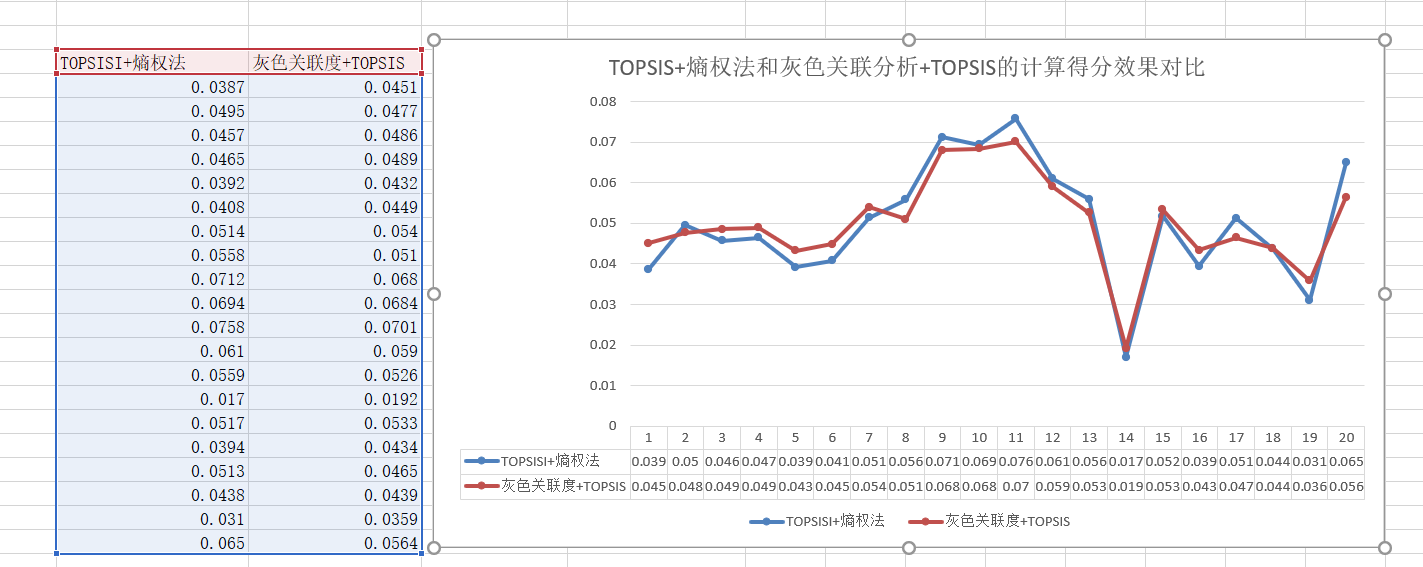总结
灰色关联分析法的优势在于，它弥补了采用数理统计方法系统分析所导致的缺憾，对样本量的多少和样本有无规律都适用，而且计算量小，十分方便，更不会出现与定性结果不符合的情况。但是灰色关联分析仅在我国有部分学者使用，在国际上并没有得到太多认可，而且当数据量较大的时候，使用标准化回归的方法是更好的选择，只有在数据很少万不得已的情况下，才考虑灰色关联分析，当然，你也可以两者综合考虑。值得一提的是，在灰色关联分析中，母序列有多个的情况，我们只需要分开计算即可，也就是说，每一个母序列，都对应一个完整的子序列。
展开全文算法
• 灰色系统理论提出了对各子系统进行灰色关联度分析的概念，意图透过一定的方法，去寻求系统中各子系统（或因素）之间的数值关系。因此，灰色关联度分析对于一个系统发展变化态势提供了量化的度量，非常适合动态历程...
• 灰色关联分析matlab代码 计算方法参考文献：王宁练：冰川平衡线变化的主导气候因子灰色关联分析 冰川冻土
• 2. 灰色关联度分析 背景： 通过对某健将级女子铅球运动员的跟踪调查，获得其 1982 年至 1986 年每年最好成绩及 16 项专项素质和身体素质的时间序列资料，见下表 ，试对此铅球运动员的专项成绩进行因素分析。 数据...
1.引言
灰色系统理论建模的主要任务是根据具体灰色系统的行为特征数据，利用不多的数据中的显信息和隐信息，寻找因素间或因素本身的数学关系
2. 灰色关联度分析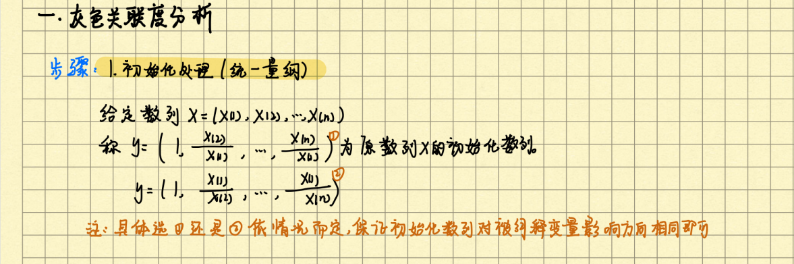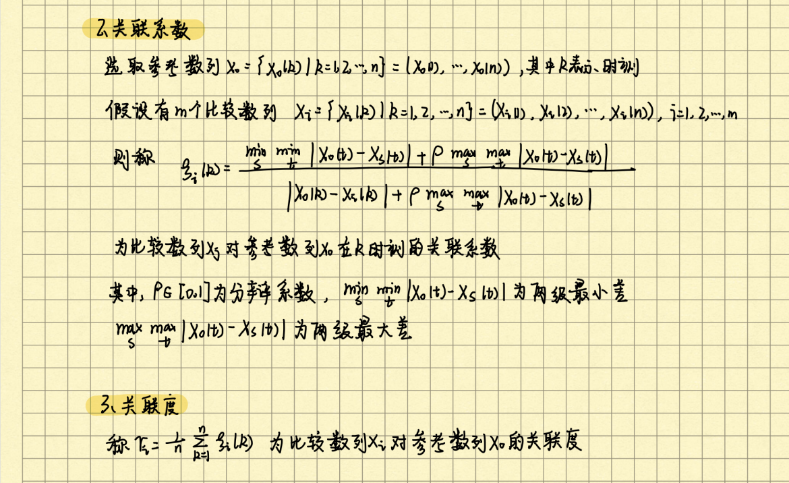背景：
通过对某健将级女子铅球运动员的跟踪调查，获得其 1982 年至 1986 年每年最好成绩及 16 项专项素质和身体素质的时间序列资料，见下表 ，试对此铅球运动员的专项成绩进行因素分析。
数据：

展开全文统计模型
• 什么是灰色关联分析 灰色关联分析是指对一个系统发展变化态势的定量描述和比较的方法，其基本思想是通过确定参考数据列和若干个比较数据列的几何形状相似程度来判断其联系是否紧密，它反映了曲线间的关联程度。 ...

什么是灰色关联分析
灰色关联分析是指对一个系统发展变化态势的定量描述和比较的方法，其基本思想是通过确定参考数据列和若干个比较数据列的几何形状相似程度来判断其联系是否紧密，它反映了曲线间的关联程度。
通常可以运用此方法来分析各个因素对于结果的影响程度，也可以运用此方法解决随时间变化的综合评价类问题，其核心是按照一定规则确立随时间变化的母序列，把各个评估对象随时间的变化作为子序列，求各个子序列与母序列的相关程度，依照相关性大小得出结论。

灰色关联分析的步骤
灰色关联分析的具体计算步骤如下：
第一步：确定分析数列。
确定反映系统行为特征的参考数列和影响系统行为的比较数列。反映系统行为特征的数据序列，称为参考数列。影响系统行为的因素组成的数据序列，称比较数列。
（1）参考数列（又称母序列）为

Y

=

Y

(

k

)

∣

k

=

1

,

2...

n

Y={Y(k) | k = 1,2...n}

；
（2）比较数列（又称子序列）为

X

i

=

X

i

(

k

)

∣

k

=

1

,

2...

n

,

i

=

1

,

2...

m

X_i={X_i(k) | k = 1,2...n},i = 1,2...m

。
第二步，变量的无量纲化
由于系统中各因素列中的数据可能因量纲不同，不便于比较或在比较时难以得到正确的结论。因此在进行灰色关联度分析时，一般都要进行数据的无量纲化处理。主要有一下两种方法 　　 （1）初值化处理：

x

i

(

k

)

=

x

i

(

k

)

x

i

(

1

)

,

k

=

1

,

2...

n

;

i

=

0

,

1

,

2...

m

x_i(k)=\frac{x_i(k)}{x_i(1)},k=1,2...n;i=0,1,2...m

（2）均值化处理：

x

i

(

k

)

=

x

i

(

k

)

x

i

ˉ

,

k

=

1

,

2...

n

;

i

=

0

,

1

,

2...

m

x_i(k)=\frac{x_i(k)}{\bar{x_i}},k=1,2...n;i=0,1,2...m

其中

k

k

对应时间段，

i

i

对应比较数列中的一行（即一个特征）
第三步，计算关联系数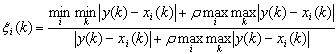记

△

i

(

k

)

=

∣

y

(

k

)

−

x

i

(

k

)

∣

\triangle_i(k)=|y(k)-x_i(k)|

，则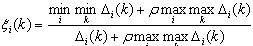ρ

∈

(

0

,

∞

)

\rho\in(0,\infty)

，称为分辨系数。ρ越小，分辨力越大，一般ρ的取值区间为

(

0

,

1

)

(0,1)

,具体取值可视情况而定。当

ρ

≤

0.5463

\rho\le0.5463

时，分辨力最好，通常取

ρ

=

0.5

ρ = 0.5

。
第四步，计算关联度
因为关联系数是比较数列与参考数列在各个时刻（即曲线中的各点）的关联程度值，所以它的数不止一个，而信息过于分散不便于进行整体性比较。因此有必要将各个时刻（即曲线中的各点）的关联系数集中为一个值，即求其平均值，作为比较数列与参考数列间关联程度的数量表示，关联度

r

i

ri

公式如下：

r

i

=

1

n

∑

k

=

1

n

ξ

i

(

k

)

,

k

=

1

,

2

,

Λ

,

n

r_i=\frac{1}{n}\sum_{k=1}^n\xi_i(k),k=1,2,\Lambda,n

第五步，关联度排序
关联度按大小排序，如果

r

1

&lt;

r

2

r1 &lt; r2

，则参考数列

y

y

与比较数列

x

2

x2

更相似。
在算出

X

i

(

k

)

Xi(k)

序列与

Y

(

k

)

Y(k)

序列的关联系数后，计算各类关联系数的平均值，平均值

r

i

ri

就称为

Y

(

k

)

Y(k)

与

X

i

(

k

)

Xi(k)

的关联度。

灰色关联分析的实例
下表为某地区国内生产总值的统计数据（以百万元计），问该地区从2000年到2005年之间哪一种产业对GDP总量影响最大。
年份国内生产总值第一产业第二产业第三产业200019883868397632001206140884680820022335422960953200327504821258101020043356511157712682005380656118931352
步骤1：确立母序列
在此需要分别将三种产业与国内生产总值比较计算其关联程度，故母序列为国内生产总值。若是解决综合评价问题时则母序列可能需要自己生成，通常选定每个指标或时间段中所有子序列中的最佳值组成的新序列为母序列。
步骤2：无量纲化处理
在此采用均值化法，即将各个序列每年的统计值与整条序列的均值作比值，可以得到如下结果：
年份国内生产总值第一产业第二产业第三产业20000.73200.83610.68280.743920010.75880.88380.68850.787820020.85970.91410.78120.929220031.01251.04401.02370.984720041.23561.10691.28331.236320051.40131.21521.54051.3182
步骤3：计算每个子序列中各项参数与母序列对应参数的关联系数其中

ξ

i

(

k

)

ξ_i(k)

表示第

i

i

个子序列的第

k

k

个参数与母序列（即0序列）的第

k

k

个参数的关联系数，$\rho\ 为 分 辨 系 数 取 值 范 围 在 为分辨系数取值范围在 [0,1]$，其取值越小求得的关联系数之间的差异性越显著，在此取为0.5进行计算可得到如下结果：
年份S01（t）S02（t）S03（t）20000.47550.65910.893320010.42990.57390.768120020.63580.54650.576720030.75270.89930.775820040.42280.66611.000020050.33580.40370.5322
步骤4：计算关联度
用公式

r

i

=

1

n

∑

k

=

1

n

ξ

i

(

k

)

,

k

=

1

,

2

,

Λ

,

n

r_i=\frac{1}{n}\sum_{k=1}^n\xi_i(k),k=1,2,\Lambda,n

， 可以得到

r

1

=

0.5088

，

r

2

=

0.6248

，

r

3

=

0.7577

r1 = 0.5088，r2 = 0.6248，r3 = 0.7577

，通过比较三个子序列与母序列的关联度可以得出结论：该地区在2000年到2005年期间的国内生产总值受到第三产业的影响最大。

灰色关联分析matlab的实现
clc;
close;
clear all;
x=x(:,2:end)';
column_num=size(x,2);
index_num=size(x,1);

% 1、数据均值化处理
x_mean=mean(x,2);
for i = 1:index_num
x(i,:) = x(i,:)/x_mean(i,1);
end
% 2、提取参考队列和比较队列
ck=x(1,:)
cp=x(2:end,:)
cp_index_num=size(cp,1);

%比较队列与参考队列相减
for j = 1:cp_index_num
t(j,:)=cp(j,:)-ck;
end
%求最大差和最小差
mmax=max(max(abs(t)))
mmin=min(min(abs(t)))
rho=0.5;
%3、求关联系数
ksi=((mmin+rho*mmax)./(abs(t)+rho*mmax))

%4、求关联度
ksi_column_num=size(ksi,2);
r=sum(ksi,2)/ksi_column_num;

%5、关联度排序，得到结果r3>r2>r1
[rs,rind]=sort(r,'descend');

运行结果：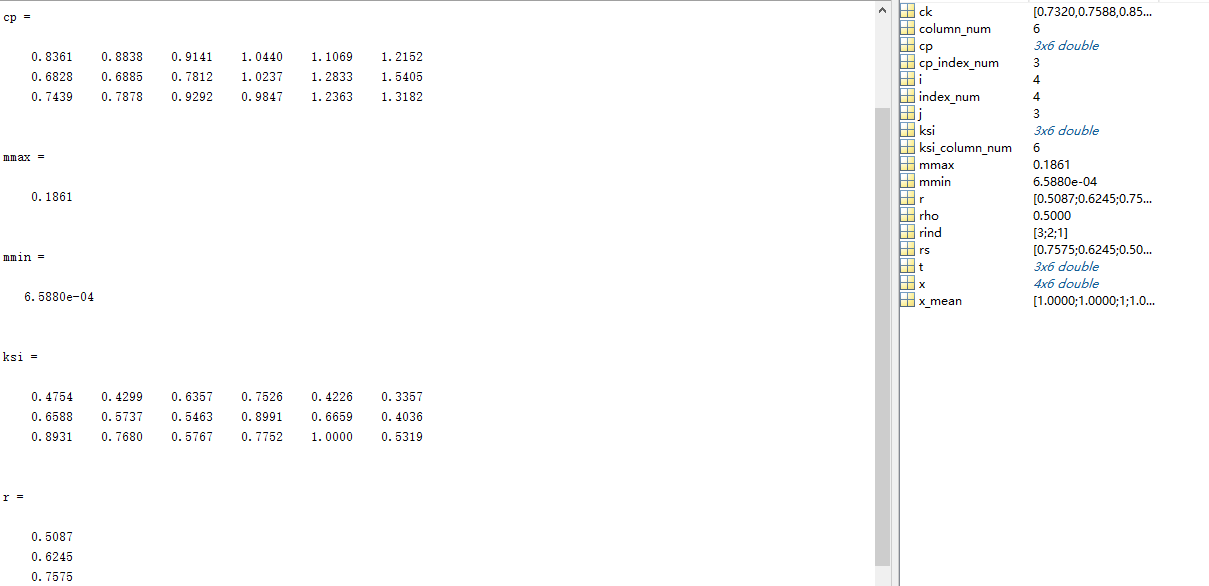灰色关联分析python的实现
import pandas as pd
x=x.iloc[:,1:].T

# 1、数据均值化处理
x_mean=x.mean(axis=1)
for i in range(x.index.size):
x.iloc[i,:] = x.iloc[i,:]/x_mean[i]

# 2、提取参考队列和比较队列
ck=x.iloc[0,:]
cp=x.iloc[1:,:]

# 比较队列与参考队列相减
t=pd.DataFrame()
for j in range(cp.index.size):
temp=pd.Series(cp.iloc[j,:]-ck)
t=t.append(temp,ignore_index=True)

#求最大差和最小差
mmax=t.abs().max().max()
mmin=t.abs().min().min()
rho=0.5
#3、求关联系数
ksi=((mmin+rho*mmax)/(abs(t)+rho*mmax))

#4、求关联度
r=ksi.sum(axis=1)/ksi.columns.size

#5、关联度排序，得到结果r3>r2>r1
result=r.sort_values(ascending=False)


展开全文python
• function [y] = relevancy( refer,compare,p,rank) %refer参考数列(行向量)，compare比较数列 %p为分辨系数，默认为0.5 %rank为列向量，反映参考数列与比较数列同...%y返回一个反映关联度的列向量 [a,b]=size(compar...
• matlab灰色关联分析法 https://blog.csdn.net/m0_37286282/article/details/79183333
• 该代码适用于数学建模中需要求权重或评估模型，只需要修改输入的数据，其余命令不变，十分简易方便
• ## 灰色关联度矩阵模型及其MATLAB实现

千次阅读 多人点赞 2019-12-06 10:41:15
灰色关联度矩阵是灰色系统另一个非常重要的领域，通常用于分析向量与向量之间或矩阵与矩阵之间的关联度，其实用性非常强。
• 对两张图片关联度分析流程图 代码 % 计算关联度 m=4; %子序列 k=15;%序列长度 X0=zeros(15,4);%差序列矩阵 R0=zeros(15,4);%关联系数矩阵 % 第一步：载入源数据 a0=[15 18 17 18 19 17 18 18 13 17 18 13 18 13 ...
• ## matlab灰色关联分析

万次阅读 多人点赞 2017-01-22 11:30:08
灰色关联分析是灰色系统理论的一个分支，应用灰色关联分析方法对受多种因素影响的事物和现象从整体观念出发进行综合评价是一个被广为接受的方法。 引一小段灰色关联分析代码，没有解释。%在归一化之前要注意因素变化...
• 灰色关联分析法，这种方法是进行关联分析的，本设计中为各指标和成绩的关联度大小排序的结果。
• 灰色关联度matlab源程序完整版 近几天一直在写算法其实网上可以下到这些算法的源程序的但是为了搞懂 搞清楚还是自己一个一个的看了写了作为自身的积累而且自己的的矩 阵计算类库也迅速得到补充以后关于算法方面基本...
• 使用matlab计算灰色关联度，直接替换数据就可以出结果
• %byallen%灰色关联分析法和灰色加权关联分析matlab程序，其区别主要在于求关联度是使用平均值法还是加权法%平均值法为一般普通求法，加权值法可以根据侧重点不同进行分析%matlab6.5%常数定义p = 0.2;%加权权值定义...
• 基于Matlab 2014b平台的灰色预测发资料和源码，可供数学建模学习使用。
• 灰色系统关联分析，横轴为时间轴，需要输入比较序列A，参考序列B，注意使用过程之中需要查看表格的转置，只能用于多对一的相关性分析，没有编多对多
• 原始数据 权重值 权重值 主函数代码： ...斜率灰色关联度算法代码 function r = relation(data) a=size(data,1); b=size(data,2); x0=data(:,1); x1=rand(a,b-1); for i=2:b x1(:,i-1)=100-250abs(x0-dat
• 灰色关联度matlab程序 我采用的初值化方法是用每一行的均值做为除数去除同一行中的其它数 我还在学习中，有错请指出呀
• 该楼层疑似违规已被系统折叠隐藏此楼查看此楼有个小问题，灰色关联度分析MATLAB代码如下：% Grey relation analysiszhuyinsu = D1(:,46);zlzdm1 = D1(:,6);zlzdwd1 = D1(:,4);zlzdc1 = D1(:,5);% define comparative...
• ## 灰色关联度分析

千次阅读 多人点赞 2019-11-13 14:46:07
灰色关联度分析 灰色关联度分析方法步骤及原理； 另附例题及代码。
• 1，灰色关联度分析（Grey Relation Analysis，GRA）原理详解 2，灰色关联分析法 3，灰色关联分析(GRA)的理论及应用（matlab和python） 4Python实现 灰色关联分析 与结果可视化 ......

# 灰色关联度分析matlabmatlab 订阅